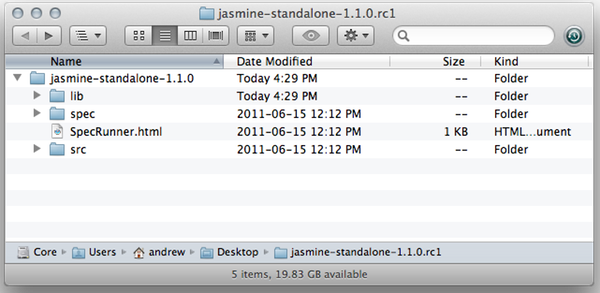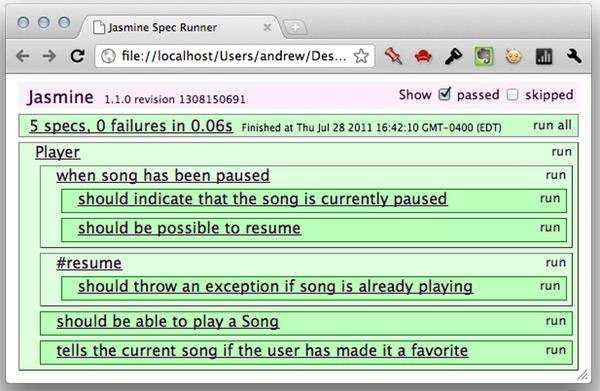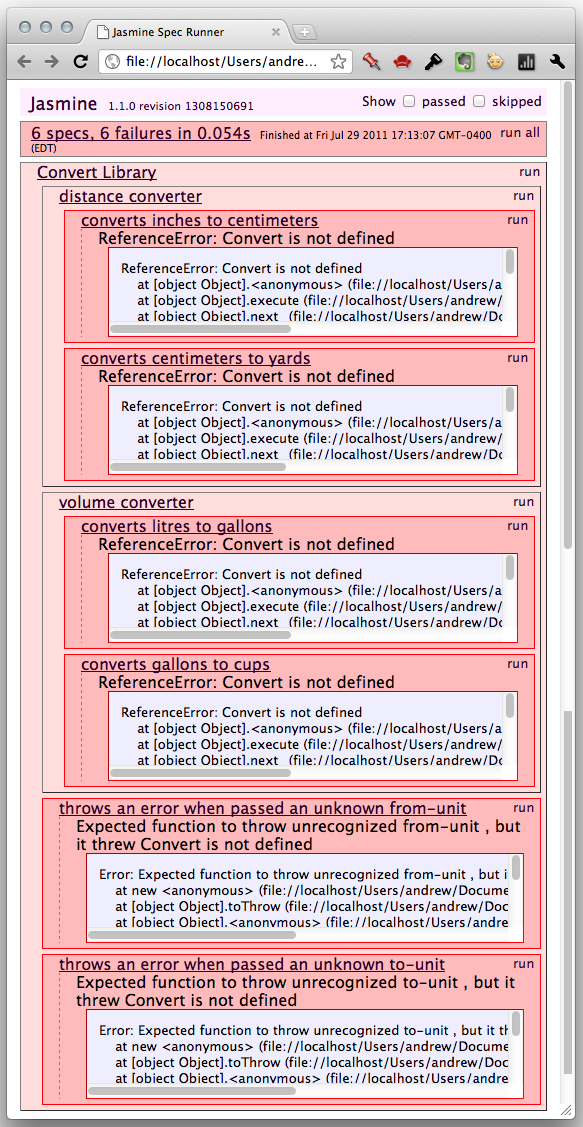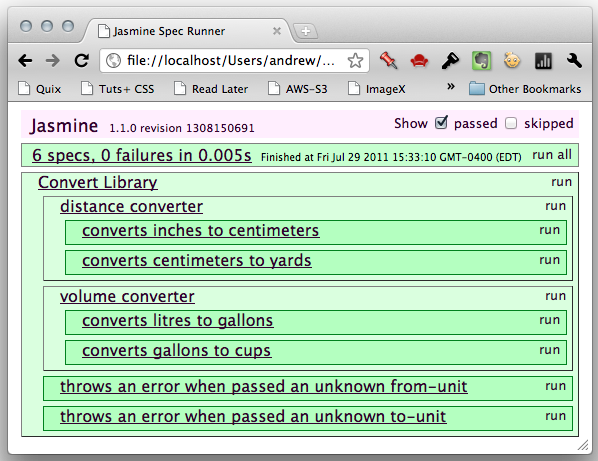# Coding Guru

### Code never does the mistake, we make the mistakes in the code. Think, Imagine, Phrase and then Code.

We all know we should be testing our code, but we don’t actually do it. I guess it’s fair to say that most of us put it off because, nine times out of ten, it means learning yet another concept. In this tutorial, I’ll introduce you to a great little framework for testing your JavaScript code with ease.

Today, we’re going to be learning about the Jasmine BDD testing framework. But we’re stopping here for a detour first, to talk very briefly, about BDD and TDD. If you’re not familiar with these acronyms, they stand for Behaviour-Driven Development and Test-Driven Development. I’m in the middle of learning about what each of these is in practice and how they are different, but here are some of the basic differences:

BDD and TDD … stand for Behaviour-Driven Development and Test-Driven Development.

TDD in its simplest form is just this:

2. Watch them fail
3. Make them pass
4. Refactor
5. Repeat

That’s pretty easy to understand, eh?

BDD is a little more complex: as I understand it right now, I don’t think that you or I as a single developer can actually practice it fully; it’s more of a team thing. Here are a few of the practices of BDD:

• Establishing the goals of different stakeholders required for a vision to be implemented
• Involving stakeholders in the implementation process through outside-in software development
• Using examples to describe the behavior of the application, or of units of code
• Automating those examples to provide quick feedback and regression testing

All this to say that, while Jasmine bills itself as a BDD framework, we’re going to be using it in a more TDD-style way. That doesn’t mean we’re using it wrong, though. Once we’re finished, you’ll be able to test your JavaScript with ease … and I expect you to do it!Jasmine takes a lot of cues from Rspec.

If you’re at all familiar with Rspec, the de facto BDD framework, you’ll see that Jasmine takes a lot of cues from Rspec. Jasmine tests are primarily two parts: `describe` blocks and `it` blocks. Let’s see how this works.

We’ll look at some closer-to-real-life tests in a few, but for now, we’ll keep it simple:

 12345 `describe(``'JavaScript addition operator'``, ``function` `() {``    ``it(``'adds two numbers together'``, ``function` `() {``        ``expect(1 + 2).toEqual(3);``    ``});``});`

Both the `describe` and `it` functions take two parameters: a text string and a function. Most test frameworks try to read as much like English as possible, and you can see this with Jasmine. First, notice that the string passed to `describe` and the string passed to `it` form a sentence (of sorts): “JavaScript addition operator adds two numbers together.” Then, we go on to show how.

Inside that `it` block, you can write all the setup code you need for your test. We don’t need any for this simple example. Once you’re ready to write the actual test code, you’ll start with the `expect` function, passing it whatever you are testing. Notice how this forms a sentence as well: we “expect 1 + 2 to equal 3.”

But I’m getting ahead of ourselves. As I said, whatever value you pass into `expect` will be tested. The method you call, off the returned value of `expect`, will be determined by which test is run. This group of methods is called ‘matchers’, and we’ll be looking at several of them today. In this case, we’re using the `toEqual` matcher, which checks to see that the value passed to `expect` and the value passed to `toEqual` are the same value.

I think you’re ready to take this to the next level, so let’s set up a simple project using Jasmine.

Jasmine can be used by itself; or you can integrate it with a Rails project. We’ll do the former. While Jasmine can run outside the browser (think Node, among other places), we can get a really nice little template with the download.You’ll find the actual Jasmine framework files in the `lib` folder. If you prefer to structure your projects differently, please do so; but we’re going to keep this for now.

There’s actually some sample code wired up in this project template. The “actual” JavaScript ( the code we want to test) can be found in the `src` subdirectory; we’ll be putting ours there shortly. The testing code—the specs—go in the `spec` folder. Don’t worry about the `SpecHelper.js` file just yet; we’ll come back to that.

That `SpecRunner.html` file is what runs the tests in a browser. Open it up (and check the “passed” checkbox in the upper right corner), and you should see something like this:This shows us that all the tests for the sample project are passing. Once you get through this tutorial, I recommend you open up the `spec/PlayerSpec.js` file and peruse that code. But right now, let’s give this test writing stuff a try.

• Create `convert.js` in the `src` folder.
• Create `convertSpec.js` in the `spec` folder,
• Copy the `SpecRunner.html` file and rename it `SpecRunner.original.html`.
• Remove the links to the sample project files in `SpecRunner.html` and add these lines:

 12 `<``script` `src``=``"src/convert.js"``><>/script>``<``script` `src``=``"spec/convertSpec.js"``>`

Now we’re ready to create a mini-library that will convert between measurement units. We’ll start by writing the tests for our mini-library.

So, let’s write our tests, shall we?

 123456789 `describe( ``"Convert library"``, ``function` `() {``    ``describe( ``"distance converter"``, ``function` `() {` `        ``});` `        ``describe( ``"volume converter"``, ``function` `() {` `    ``});``});`

We start with this; we’re testing our `Convert` library. You’ll notice that we’re nesting `describe` statements here. This is perfectly legal. It’s actually a great way to test seperate functionality chunks of the same codebase. Instead of two seperate `describe` calls for `Convert` library’s distance conversions and volume conversions, we can have a more descriptive suite of tests like this.

Now, onto the actual tests. I’ll repeat the inner `describe` calls here for your convenience.

 123456789 `describe( ``"distance converter"``, ``function` `() {``    ``it(``"converts inches to centimeters"``, ``function` `() {``        ``expect(Convert(12, ``"in"``).to(``"cm"``)).toEqual(30.48);``    ``});` `    ``it(``"converts centimeters to yards"``, ``function` `() {``        ``expect(Convert(2000, ``"cm"``).to(``"yards"``)).toEqual(21.87);``    ``});``});`

Here are our tests for distance conversions. It’s imporant to notice something here: we haven’t written a speck of code for our `Convert` library yet, so in these tests we’re doing more than just check to see if it works: we’re actually deciding how it will be used (and therefore implemented). Here’s how we’ve decided to make our conversions:

 1 `Convert(, ).to();`

Yes, I’m taking a cue from the way Jasmine has implemented its tests, but I think it’s a nice format. So, in these two tests, I’ve made the conversions myself (ok, with a calculator) to see what the results of our calls should be. We’re using the `toEqual` matcher to see if our tests pass.

Here’s the volume tests:

 123456789 `describe( ``"volume converter"``, ``function` `() {``    ``it(``"converts litres to gallons"``, ``function` `() {``        ``expect(Convert(3, ``"litres"``).to(``"gallons"``)).toEqual(0.79);``    ``});` `    ``it(``"converts gallons to cups"``, ``function` `() {``        ``expect(Convert(2, ``"gallons"``).to(``"cups"``)).toEqual(32);``    ``});``});`

And I’m going to add two more tests in our top-level `describe` call:

 01020304050607080910111213 `it(``"throws an error when passed an unknown from-unit"``, ``function` `() {``    ``var` `testFn = ``function` `() {``        ``Convert(1, ``"dollar"``).to(``"yens"``);``    ``}``    ``expect(testFn).toThrow(``new` `Error(``"unrecognized from-unit"``));``});` `it(``"throws an error when passed an unknown to-unit"``, ``function` `() {``    ``var` `testFn = ``function` `() {``        ``Convert(1, ``"cm"``).to(``"furlongs"``);``    ``}``    ``expect(testFn).toThrow(``new` `Error(``"unrecognized to-unit"``));``});`

These check for errors that should be thrown when unknown units are passed into either the `Convert` function or the `to` method. You’ll notice that I’m wrapping the actual conversion in a function and passing that to the `expect` function. That’s because we can’t call the function as the `expect` parameter; we need to hand it a function and let it call the function itself. Since we need to pass a parameter to that `to` function, we can do it this way.

The other thing to note is that I’m introducing a new matcher: `toThrow`, which takes an error object. We’ll look at some more matchers soon.

Now, if you open `SpecRunner.html` in a browser, you’ll get this:Great! Our tests are failing. Now, let’s open our `convert.js` file and do some work:

 0102030405060708091011121314151617181920212223242526272829303132333435363738394041424344454647 `function` `Convert(number, fromUnit) {``    ``var` `conversions = {``            ``distance : {``                ``meters : 1,``                ``cm     : 0.01,``                ``feet   : 0.3048,``                ``inches : 0.0254,``                ``yards  : 0.9144``            ``},``            ``volume : {``                ``liters : 1,``                ``gallons: 3.785411784,``                ``cups   : 0.236588236 ``            ``}``        ``},``        ``betweenUnit = ``false``,``        ``type, unit;` `    ``for` `(type ``in` `conversions) {``        ``if` `(conversions(type)) {``            ``if` `( (unit = conversions[type][fromUnit]) ) {``                ``betweenUnit = number * unit * 1000;``            ``}``        ``}``    ``}` `    ``return` `{``        ``to : ``function` `(toUnit) {``            ``if` `(betweenUnit) {``                ``for` `(type ``in` `conversions) {``                    ``if` `(conversions.hasOwnProperty(type)) {``                        ``if` `( (unit = conversions[type][toUnit]) ) {``                            ``return` `fix(betweenUnit / (unit * 1000));``                        ``}``                    ``}``                ``}``                ``throw` `new` `Error(``"unrecognized to-unit"``);``            ``} ``else` `{``                ``throw` `new` `Error(``"unrecognized from-unit"``);``            ``}  ` `            ``function` `fix (num) {``                ``return` `parseFloat( num.toFixed(2) );``            ``}``        ``}``    ``};``}`

We’re not really going to discuss this, because we’re learning Jasmine here. But here are the main points:

• We’re making the conversions by storing the conversion in an object; conversion numbers are classified by type (distance, volume, add your own). For each field of measurement, we have a base value (meters or liters, here) that everything converts to. So when you see `yards: 0.9144`, you know that that’s how many yards there are in a meter. Then, to convert yards to, say, centimeters, we multiply `yards` by the first parameter (to get the number of meters) and then divide the product by `cm`, the number of meters in a centimeter. This way, we don’t have to store the conversion rates for every pair of values. This also makes it easy to add new values later.
• In our case, we’re expecting the units passed in to be the same as the keys we’re using in the conversion “table.” If this were a real library, we’d want to support multiple formats—like ‘in’, ‘inch’, and ‘inches’—and therefore we’d want to add some logic to match the `fromUnit` to the right key.
• At the end of the `Convert` function, we store the intermediate value in `betweenUnit`, which is initialized to `false`. That way, if we don’t have the `fromUnit`, `betweenUnit` will be `false` going into the `to` method, and so an error with be thrown.
• If we don’t have the `toUnit`, a different error will be thrown. Otherwise, we’ll divide as neccessary and return the converted value.

Now, go back to `SpecRunner.html` and reload the page. You should now see this (after checking “Show passed”):There you go! Our tests are passing. If we were developing a real project here, we would write tests for a certain chunk of functionality, make them pass, write tests for another check, make them passs, etc. But since this was a simple example, we’ve just done it all in one fell swoop.

And now that you’ve seen this simple example of using Jasmine, let’s look at a few more features that it offers you.

So far, we’ve used two matchers: `toEqual` and `toThrow`. There are, of course, many others. Here are a few you’ll probably find useful; you can see the whole list on the wiki.

If you just want to make sure a variable or property is defined, there’s a matcher for that. There’s also one to confirm that a variable or property is `undefined`.

 123456789 `it(``"is defined"``, ``function` `() {``    ``var` `name = ``"Andrew"``;``    ``expect(name).toBeDefined();``})` `it(``"is not defined"``, ``function` `() {``    ``var` `name;``    ``expect(name).toBeUndefined();``});`

If something should be true or false, these matchers will do it.

 123456 `it(``"is true"``, ``function` `() {``    ``expect(Lib.isAWeekDay()).toBeTruthy();``});``it(``"is false"``, ``function` `() {``    ``expect(Lib.finishedQuiz).toBeFalsy();``});`

For all you number people. You know how these work:

 123456 `it(``"is less than 10"``, ``function` `() {``    ``expect(5).toBeLessThan(10);``});``it(``"is greater than 10"``, ``function` `() {``    ``expect(20).toBeGreaterThan(10);``});`

Have some output text that should match a regular expression? The `toMatch` matcher is ready and willing.

 123 `it(``"outputs the right text"``, ``function` `() {``    ``expect(cart.total()).toMatch(/\\$\d*.\d\d/);``});`

This one is pretty useful. It checks to see if an array or string contains an item or substring.

 123 `it(``"should contain oranges"``, ``function` `() {``    ``expect([``"apples"``, ``"oranges"``, ``"pears"``]).toContain(``"oranges"``);``});`

There are a few other matchers, too, that you can find in the wiki. But what if you want a matcher that doesn’t exist? Really, you should be able to do just about anything with some set-up code and the matchers Jasmine provides, but sometimes it’s nicer to abstract some of that logic to have a more readable test. Serendipitously (well, actually not), Jasmine allows us to create our own matchers. But to do this, we’ll need to learn a little something else first.

Often—when testing a code base—you’ll want to perform a few lines of set-up code for every test in a series. It would be painful and verbose to have to copy that for every `it` call, so Jasmine has a handy little feature that allows us to designate code to run before or after each test. Let’s see how this works:

 01020304050607080910111213141516 `describe(``"MyObject"``, ``function` `() {``    ``var` `obj = ``new` `MyObject();` `    ``beforeEach(``function` `() {``        ``obj.setState(``"clean"``);``    ``});` `    ``it(``"changes state"``, ``function` `() {``        ``obj.setState(``"dirty"``);``        ``expect(obj.getState()).toEqual(``"dirty"``);``    ``})``    ``it(``"adds states"``, ``function` `() {``        ``obj.addState(``"packaged"``);``        ``expect(obj.getState()).toEqual([``"clean"``, ``"packaged"``]);``    ``})``});`

In this contrived example, you can see how, before each test is run, the state of `obj` is set to “clean”. If we didn’t do this, the changed made to an object in a previous test persist to the next test by default. Of course, we could also do something similar with the `AfterEach` function:

 01020304050607080910111213141516 `describe(``"MyObject"``, ``function` `() {``    ``var` `obj = ``new` `MyObject(``"clean"``); ``// sets initial state` `    ``afterEach(``function` `() {``        ``obj.setState(``"clean"``);``    ``});` `    ``it(``"changes state"``, ``function` `() {``        ``obj.setState(``"dirty"``);``        ``expect(obj.getState()).toEqual(``"dirty"``);``    ``})``    ``it(``"adds states"``, ``function` `() {``        ``obj.addState(``"packaged"``);``        ``expect(obj.getState()).toEqual([``"clean"``, ``"packaged"``]);``    ``})``});`

Here, we’re setting up the object to begin with, and then having it corrected after every test. If you want the `MyObject` function so you can give this code a try, you can get it here in a GitHub gist.

Like we said earlier, customer matchers would probably be helpful at times. So let’s write one. We can add a matcher in either a `BeforeEach` call or an `it` call (well, I guess you could do it in an `AfterEach` call, but that wouldn’t make much sense). Here’s how you start:

 12345 `beforeEach(``function` `() {``    ``this``.addMatchers({` `    ``});``});`

Pretty simple, eh? We call `this.addMatchers`, passing it an object parameter. Every key in this object will become a matcher’s name, and the associated function (the value) will be how it is run. Let’s say we want to create a matcher that with check to see if one number is between two others. Here’s what you’d write:

 010203040506070809101112 `beforeEach(``function` `() {``    ``this``.addMatchers({``        ``toBeBetween: ``function` `(rangeFloor, rangeCeiling) {``            ``if` `(rangeFloor > rangeCeiling) {``                ``var` `temp = rangeFloor;``                ``rangeFloor = rangeCeiling;``                ``rangeCeiling = temp;``            ``}``            ``return` `this``.actual > rangeFloor && ``this``.actual < rangeCeiling;``        ``}``    ``});``});`

We simply take two parameters, make sure the first one is smaller than the second, and return a boolean statement that evaluates to true if our conditions are met. The important thing to notice here is how we get a hold of the value that was passed to the `expect` function: `this.actual`.

 1234567 `it(``"is between 5 and 30"``, ``function` `() {``    ``expect(10).toBeBetween(5, 30);``});` `it(``"is between 30 and 500"``, ``function` `() {``    ``expect(100).toBeBetween(500, 30);``});`

This is what the `SpecHelper.js` file does; it has a `beforeEach` call that adds the matcher `tobePlaying()`. Check it out!

There’s a lot more you can do with Jasmine: function-related matchers, spies, asynchronous specs, and more. I recommend you explore the wiki if you’re interested. There are also a few accompanying libraries that make testing in the DOM easier: Jasmine-jQuery, and Jasmine-fixture (which depends on Jasmine-jQuery).

So if you aren’t testing your JavaScript so far, now is an excellent time to start. As we’ve seen, Jasmine's fast and simple syntax makes testing pretty simple. There’s just no reason for you not to do it, now, is there?